## Example Questions

### Example Question #2 : How To Find The Solution To A Quadratic Equation

What is the sum of the values of x that satisfy the following equation:

16x – 10(4)x + 16 = 0.

5/2

2

3/2

1

4

2

Explanation:

The equation we are asked to solve is 16x – 10(4)x + 16 = 0.

Equations of this type can often be "transformed" into other equations, such as linear or quadratic equations, if we rewrite some of the terms.

First, we can notice that 16 = 42. Thus, we can write 16x as (42)x or as (4x)2.

Now, the equation is (4x)2 – 10(4)x + 16 = 0

Let's introduce the variable u, and set it equal 4x. The advantage of this is that it allows us to "transform" the original equation into a quadratic equation.

u2 – 10u + 16 = 0

This is an equation with which we are much more familiar. In order to solve it, we need to factor it and set each factor equal to zero. In order to factor it, we must think of two numbers that multiply to give us 16 and add to give us –10. These two numbers are –8 and –2. Thus, we can factor u2 – 10u + 16 = 0 as follows:

(u – 8)(u – 2) = 0

Next, we set each factor equal to 0.

u – 8 = 0

u = 8

u – 2 = 0

u = 2.

Thus, u must equal 2 or 8. However, we want to find x, not u. Since we defined u as equal to 4x, the equations become:

4x = 2 or 4x = 8

Let's solve 4x = 2 first. We can rewrite 4x as (22)x = 22x, so that the bases are the same.

22x = 2 = 21

2x = 1

x = 1/2

Finally, we will solve 4x = 8. Once again, let's write 4x as 22x. We can also write 8 as 23.

22x = 23

2x = 3

x = 3/2

The original question asks us to find the sum of the values of x that solve the equation. Because x can be 1/2 or 3/2, the sum of 1/2 and 3/2 is 2.

### Example Question #5 : How To Find The Solution To A Quadratic Equation

I. real

II. rational

III. distinct

Which of the descriptions characterizes the solutions of the equation 2x2 – 6x + 3 = 0?

II only

I and II only

I only

I and III only

II and III only

I and III only

Explanation:

The equation in the problem is quadratic, so we can use the quadratic formula to solve it. If an equation is in the form ax2 + bx + c = 0, where a, b, and c are constants, then the quadratic formula, given below, gives us the solutions of x.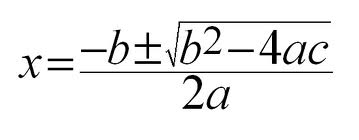In this particular problem, a = 2, b = –6, and c = 3.

The value under the square-root, b– 4ac, is called the discriminant, and it gives us important information about the nature of the solutions of a quadratic equation.

If the discriminant is less than zero, then the roots are not real, because we would be forced to take the square root of a negative number, which yields an imaginary result. The discriminant of the equation we are given is (–6)2 – 4(2)(3) = 36 – 24 = 12 > 0. Because the discriminant is not negative, the solutions to the equation will be real. Thus, option I is correct.

The discriminant can also tell us whether the solutions of an equation are rational or not. If we take the square root of the discriminant and get a rational number, then the solutions of the equation must be rational. In this problem, we would need to take the square root of 12. However, 12 is not a perfect square, so taking its square root would produce an irrational number. Therefore, the solutions to the equation in the problem cannot be rational. This means that choice II is incorrect.

Lastly, the discriminant tells us if the roots to an equation are distinct (different from one another). If the discriminant is equal to zero, then the solutions of x become (–b + 0)/2a and (–b – 0)/2a, because the square root of zero is 0. Notice that (–b + 0)/2a is the same as (–b – 0)/2a. Thus, if the discriminant is zero, then the roots of the equation are the same, i.e. indistinct. In this particular problem, the discriminant = 12, which doesn't equal zero. This means that the two roots will be different, i.e. distinct. Therefore, choice III applies.

The answer is choices I and III only.

### Example Question #6 : How To Find The Solution To A Quadratic Equation

Solve for x.

3x2 + 15x – 18 = 0.

x = 6 or x = –1

x = 5 or x = 1

x = –6 or x = 1

x = –2 or x = 3

x = 2 or x = –3

x = –6 or x = 1

Explanation:

First let's see if there is a common term.

3x2 + 15x – 18 = 0

We can pull out a 3: 3(x2 + 5x – 6) = 0

Divide both sides by 3: x2 + 5x – 6 = 0

We need two numbers that sum to 5 and multiply to –6. 6 and –1 work.

(x + 6)(x – 1) = 0

x = –6 or x = 1

### Example Question #21 : How To Find The Solution To A Quadratic Equation

The expression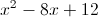is equal to 0 when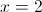and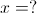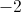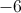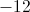Explanation:

Factor the expression and set each factor equal to 0: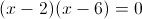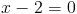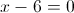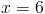### Example Question #11 : How To Find The Solution To A Quadratic Equation

Two positive consecutive multiples of four have a product of 96.  What is the sum of the two numbers?Explanation:

Let= the first number and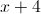= the second number.

So the equation to solve becomes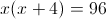.  This quadratic equation needs to be multiplied out and set equal to zero before factoring.  Then set each factor equal to zero and solve.  Only positive numbers are correct, so the answer is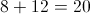.

### Example Question #23 : How To Find The Solution To A Quadratic Equation

Two consecutive positive odd numbers have a product of 35.  What is the sum of the two numbers?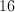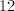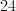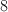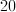Explanation:

Let= first positive number and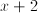= second positive number.

The equation to solve becomes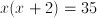We multiply out this quadratic equation and set it equal to 0, then factor.

### Example Question #24 : How To Find The Solution To A Quadratic Equation

The product of two consecutive positive multiples of four is 192.  What is the sum of the two numbers?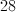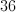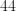Explanation:

Let= the first positive number and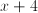= the second positive number

The equation to solve becomes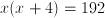We solve this quadratic equation by multiplying it out and setting it equal to 0.  The next step is to factor.

### Example Question #31 : How To Find The Solution To A Quadratic Equation

Two consecutive positive multiples of three have a product of 504.  What is the sum of the two numbers?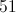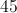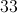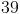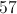Explanation:

Let= the first positive number and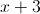= the second positive number.

So the equation to solve is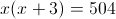We multiply out the equation and set it equal to zero before factoring.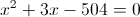thus the two numbers are 21 and 24 for a sum of 45.

### Example Question #32 : How To Find The Solution To A Quadratic Equation

Two consecutive positive numbers have a product of 420.  What is the sum of the two numbers?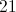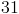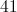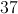Explanation:

Let= first positive number and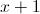= second positive number

So the equation to solve becomes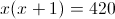Using the distributive property, multiply out the equation and then set it equal to 0.  Next factor to solve the quadratic.

### Example Question #33 : How To Find The Solution To A Quadratic Equation

A rectangle has a perimeter of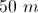and an area of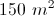What is the difference between the length and width?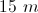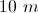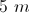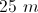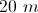Explanation:

For a rectangle,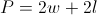and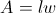where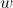= width and= length.

So we get two equations with two unknowns: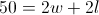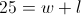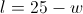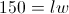Making a substitution we get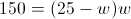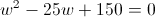Solving the quadratic equation we get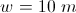or.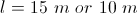The difference is.

### All ACT Math Resources